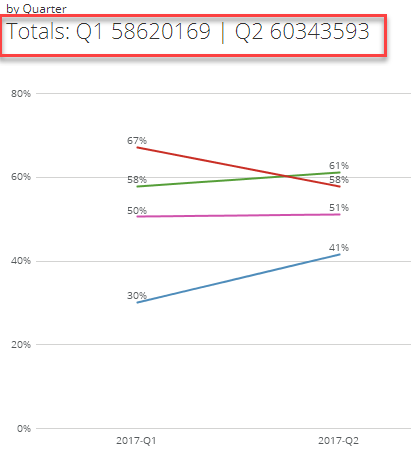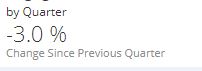# Need Help With Beast Mode| Percentage Change From Previous Quarter

I need help in fixing below code. Current code is getting the value of totals of both quarters:CONCAT(

-- This portion can be updated to include whatever text or formulas needed

'Totals: Q1 '

,SUM(CASE  WHEN (MONTH(`Date`) IN (11,12) AND YEAR(`Date`) = 2016) OR (MONTH(`Date`) = 1 AND YEAR(`Date`) = 2017) THEN `Unique Visitors` END )

,' | Q2 '

,SUM(CASE  WHEN (MONTH(`Date`) IN (2,3,4) AND YEAR(`Date`) = 2017) THEN `Unique Visitors` END)

)

But I need to display % change from previous quarter (something like in below screenshot):